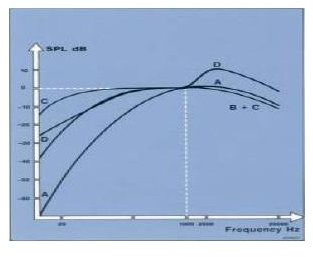# How do we measure Sound?

Let me start this article with a basic question, why should we measure sound?

To know more about something we need to measure them, this not only enables us to understand a particular criterion better but also helps us to device methodologies enabling us to control the same. The same applies to sound.

The measurement of sound will help us to precisely and scientifically control the unwanted sounds. Moreover the intensity of sound tends to play a very important role in ones hearing capability. Exposure to high levels of sound can cause damage to the ears and its sensitivity to hear. This is done separately in a field of study known as audiometry.

The various methods by which we measure sound are as follows:

• Sound Pressure Level
• Sound Intensity Level
• Sound Power Level
• Frequency Spectrum
• Frequency response function.

Sound Pressure Level

Sound is basically a pressure variation in a medium that our human ear detects. Well then the basic instrument that we use for the measurement of pressure is a barometer. A barometer is not very quick in measuring pressure variations, and take the case of sound the minimum amount of variations what we tend to hear is about 20 per second, hence the barometer is not a viable option for measurement of sound.

This led to the invention of a device that is capable of measuring the variations in the sound pressure at very quick intervals. This device is known as a sound level meter. The unit by which we measure the sound pressure level is dB(decibels).

The decibel is basically a ratio between a measured quantity and an agreed reference level of sound. The Decibel scale is logarithmic in nature. Our hearing capability varies from 0 dB(A) to 130 db(A), where 0 dB(A) is the threshold of what we can hear and 130 db(A) is the threshold of what we can bear.

Moreover by now you must me quite versatile with the term Decibels, but hope you have noticed the term (A) next to the Decibels symbol. What does this (A) mean?

Scales of Measurement of Ssound

There are actually 4 scales of measurement of sound in decibels. The scales are A, B, C and D. These scales we determined based on the necessity to measure the sound levels the same way as our human ear hears. These scales formed a basis on how the electronic measurement systems used for measuring sound should work.

A small figure is attached herewith for you just to understand how these scales look like.The scale (A) nearly matches the same way how we perceive the sounds, hence this scale is found to be more used in all literatures available. The scales ‘B’ and ‘C’ are used in areas where the pressure fluctuations tend to be more than the human hearing range. The scale ‘D’ has been specifically developed and standardized for the aircraft noise measurement.

## This post is part of the series: Sound Intensity Measurement / Measurment of Sound Intensity

Sound Intensity Measurement / Measurment of Sound Intensity